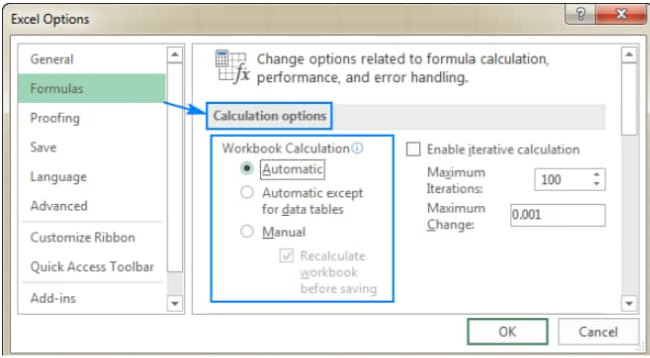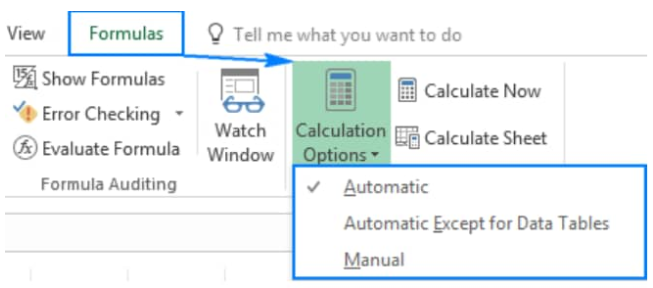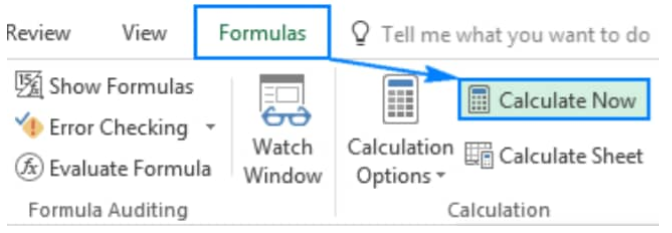Get instant live expert help with Excel or Google Sheets“My Excelchat expert helped me in less than 20 minutes, saving me what would have been 5 hours of work!”

#### Post your problem and you’ll get Expert help in seconds.

Your message must be at least 40 characters
Our professional Expert are available now. Your privacy is guaranteed.

# How to Auto calculate in Excel

The automatic calculator in Excel controls when and how formulas are recalculated.

By default, the auto recalculate Excel feature is always ON, ensuring that any formulas we enter into our worksheet gets recalculated immediately when we open our worksheet or make any changes in names or data sets on which our formulas depend.Figure 1. Calculation Options in Excel

To use automatic calculations in Excel efficiently, there are 3 essential considerations that we need to understand;

• Calculation – This is the procedure for computing formulas as well as displaying the resulting values in those cells that contain said formulas.

To prevent unnecessary calculations which can waste our time and reduce the speed of our computer, Microsoft Excel will recalculate formulas automatically only when the data in those cells which the formulas depend on has changed.

This is the go to behavior when we first open our worksheet and when we are editing the worksheet. However, we can regulate when and how automatic recalculation in Excel takes place.

• Iteration – This refers to how worksheets are repeatedly recalculated until a specified numeric condition has been met.

Excel auto calculate cannot work for a formula which refers to the same cell — whether directly or indirectly — that contains our formula. This is known as a “circular reference”.

If our formula is referring back to its own cell(s), we must determine the number of times such formulas should recalculate.

Circular references are able to iterate indefinitely. Still, we can regulate the maximum amount of iterations as well as the frequency of acceptable changes.

• Precision – This refers to the degree of accuracy of an Excel calculation.

Excel calculates and stores  with 15 important digits of precision

Yet, we can regulate the precision of these calculations to ensure that Excel makes use of the displayed values rather than the stored values whenever Excel recalculates formulas.

## How to Auto Calculate in Excel

Our worksheet has calculation options that allow us to determine when and how auto calc for Excel formulas takes place.

When we first open/edit our worksheet, the auto calculator in Excel immediately recalculates any formulas whose dependent cell, or formula values have changed.

However, we are free to change this behavior to stop Excel calculations.

To modify Excel calculation options;

1. On our worksheet ribbon, click on the “Formulas” tab and then. Under the “Calculation” group, click on “Calculation Options” and select any of the available options:Figure 2. of Calculation Options in Excel

“Automatic” is the default option. It instructs Excel to recalculate any dependent formulas automatically each time any information referenced in our worksheet cells is changed.

The “Automatic Except for Data Tables” option instructs auto calc Excel to automatically recalculate any dependent formulas excluding data tables.

Note however, that this option will turn off calculations in Excel for data tables only, meanwhile the regular Excel table will execute automatic calculations in Excel.

Enabling the “Manual” option will turn off calculations in Excel. Open worksheets will only be recalculated when we force Excel to recalculate.

## How to Force Excel to Recalculate

When we open a worksheet and excel is not recalculating our imputed formulas, it most likely means that Excel auto calculate is OFF.

We can either enable the automatic calculator in Excel (see figure 2. above), or force Excel to recalculate.

To recalculate manually, open our worksheet, update all data values, and then click on the “Formulas” tab > “Calculation” group, and then click on the “Calculate Now” button;Figure 3. Calculate Now Button in Excel

## Instant Connection to an Excel Expert

Most of the time, the problem you will need to solve will be more complex than a simple application of a formula or function. If you want to save hours of research and frustration, try our live Excelchat service! Our Excel Experts are available 24/7 to answer any Excel question you may have. We guarantee a connection within 30 seconds and a customized solution within 20 minutes.